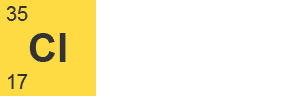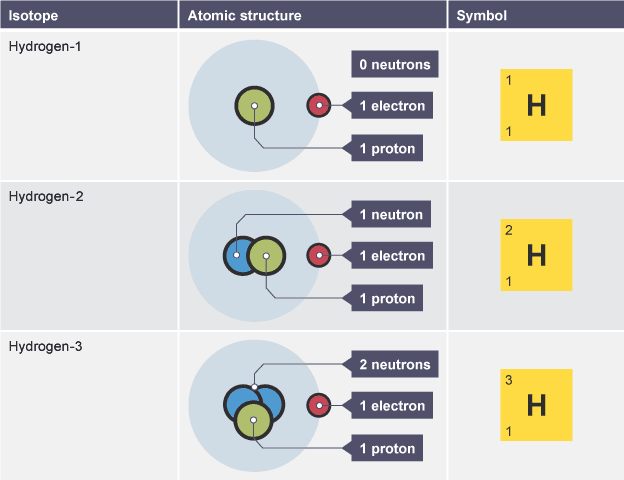# Group 1 - the alkali metals

?

Atoms contain three sub-atomic particles called protonsneutronsand electrons.

The protons and neutrons are found in the nucleus at the centre of the atom. The nucleus is very much smaller than the atom as a whole. The electrons are arranged in shells around the nucleus.

Proton 1 +1 Neutron 1 0 Electron-1

The number of electrons in an atom is always the same as the number of protons, so atoms are electrically neutral overall.

• if an atom loses one or mor
• if an atom gains one or more electrons, it becomes a negatively charged ion
• e electrons, it becomes The number of protons in the nucleus of an atom is called its atomic number:
• the atoms of a particular element all have the same number of protons
• the atoms of different elements have different numbers of protons

The total number of protons and neutrons in an atom is called its mass number.Chemical symbol of chlorine-35

The proton number is shown below the chemical symbol, and the mass number is shown above. In this example the atomic number is 17 and the mass number is 35. This means that each of these atoms has a positively charged ionDifferent atoms have different masses. Atoms have such a small mass it is more convenient to know their masses compared to each other. Carbon is taken as the standard atom and has a relative atomic mass (Ar) of 12.
• Atoms with an Ar of less than this have a smaller mass than a carbon atom.
• Atoms with an Ar that is more than this have a larger mass than a carbon atom.

# Ar values of elements

The table shows some Ar values:

ElementRelative atomic mass Hydrogen (H) 1 Carbon (C) 12 Oxygen (O) 16 Magnesium (Mg) 24 Chlorine (Cl) 35.5

These values tell you that a magnesium atom has twice the mass of a carbon atom, and 24 times more mass than a hydrogen atom. They also tell you that hydrogen atoms have 12 times less mass than a carbon atom. The Ar values also allow you to work out that three oxygen atoms have the same mass as two magnesium atoms.

Chlorine's Ar of 35.5 is an average of the masses of the different isotopes of chlorine.

# Calculating relative atomic mass from isotopic abundance

The relative atomic mass of an element is a weighted average of the masses of the atoms of the isotopes - because if there is much more of one isotope then that will influence the average mass much more than the less abundant isotope will.

For example, chlorine has two isotopes: 35Cl and 37Cl. But the relative atomic mass of chlorine is not 36. In any sample of chlorine, 75 per cent of the atoms are 35Cl and the remaining 25 per cent are 37Cl.